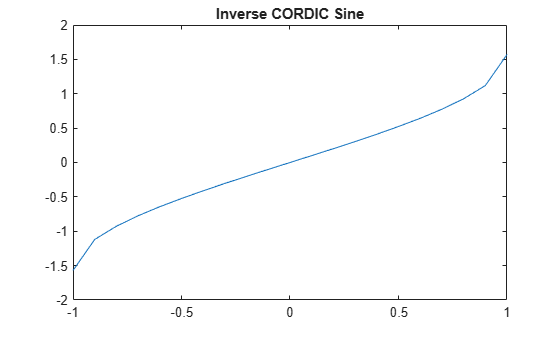# cordicasin

CORDIC-based approximation of inverse sine

## Syntax

``theta = cordicasin(x)``
``theta = cordicasin(x, niters)``

## Description

example

````theta = cordicasin(x)` returns the inverse sine of `x` based on a CORDIC approximation.```

example

````theta = cordicasin(x, niters)` returns the inverse sine of `x` performing `niters` iterations of the CORDIC algorithm.```

## Examples

collapse all

Compute the inverse Sine of a fixed-point `fi` object using a CORDIC implementation.

```a = fi(-1:.1:1,1,16); b = cordicasin(a); plot(a, b); title('Inverse CORDIC Sine');```Find the inverse sine of a `fi` object using a CORDIC implementation and specify the number of iterations the CORDIC kernel should perform. Plot the CORDIC approximation of the inverse sine with varying numbers of iterations.

```a = fi(-1:.1:1, 1, 16); for i = 5:5:20 b = cordicasin(a,i); plot(a,b); hold on; end legend('5 iterations', '10 iterations', '15 iterations', '20 iterations')```## Input Arguments

collapse all

Numeric input, specified as a scalar, vector, matrix, or multidimensional array.

Data Types: `single` | `double` | `int8` | `int16` | `int32` | `int64` | `uint8` | `uint16` | `uint32` | `uint64` | `fi`
Complex Number Support: Yes

The number of iterations that the CORDIC algorithm performs, specified as a positive, integer-valued scalar. If you do not specify `niters`, the algorithm uses a default value. For fixed-point inputs, the default value of `niters` is one less than the word length of the input array, `theta`. For double-precision inputs, the default value of `niters` is 52. For single-precision inputs, the default value is 23.

Data Types: `single` | `double` | `int8` | `int16` | `int32` | `int64` | `uint8` | `uint16` | `uint32` | `uint64` | `fi`

## Output Arguments

collapse all

Inverse sine angle values in rad.

### Functions

Introduced in R2018b

## SupportGet trial now